1. Obtain the Nash equilibrium for the following games using backward induction: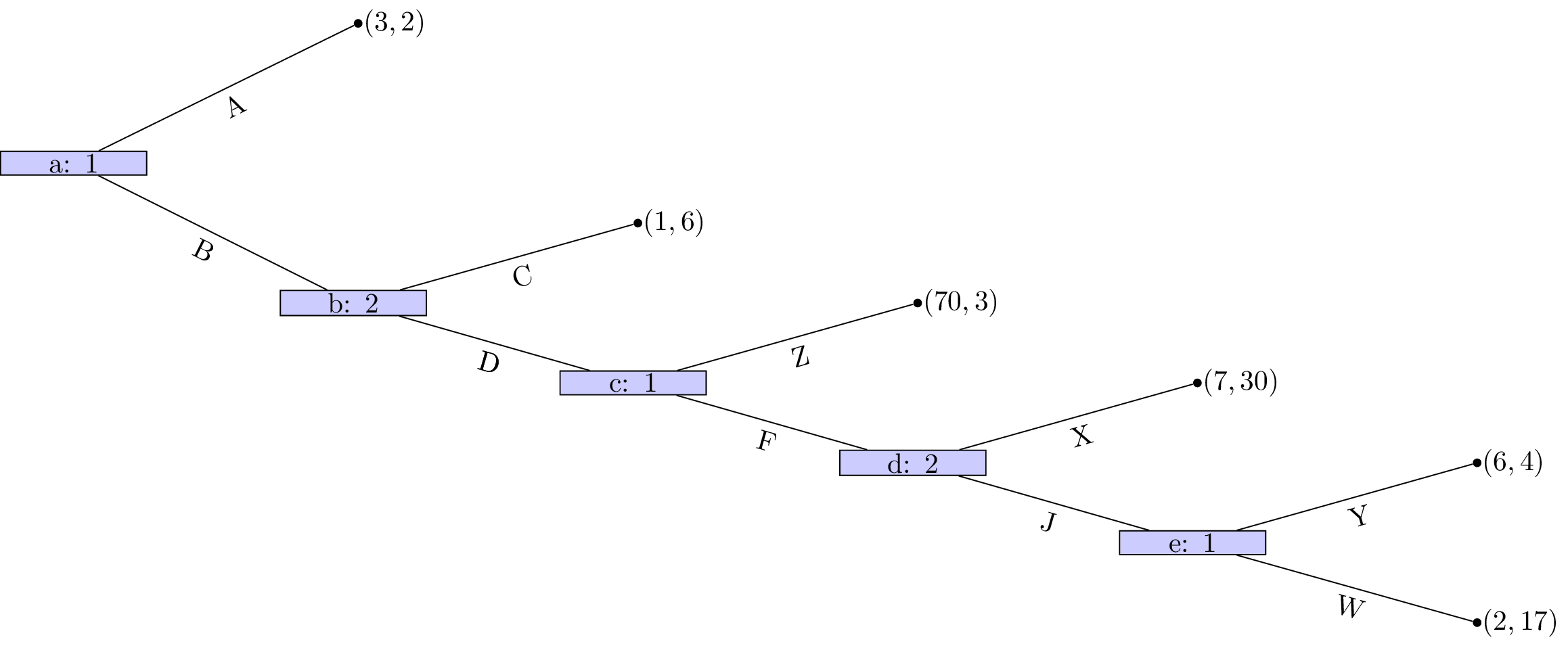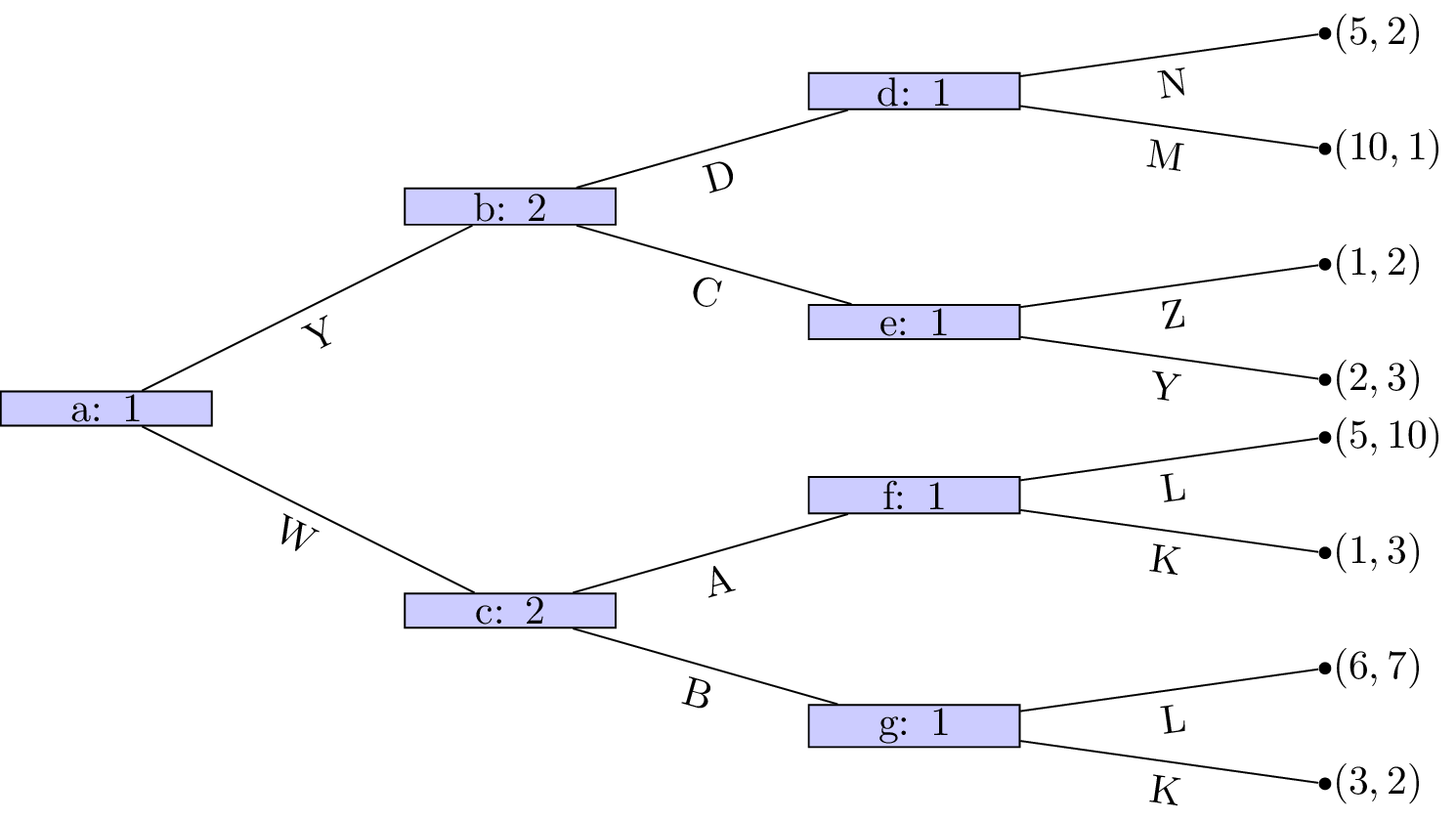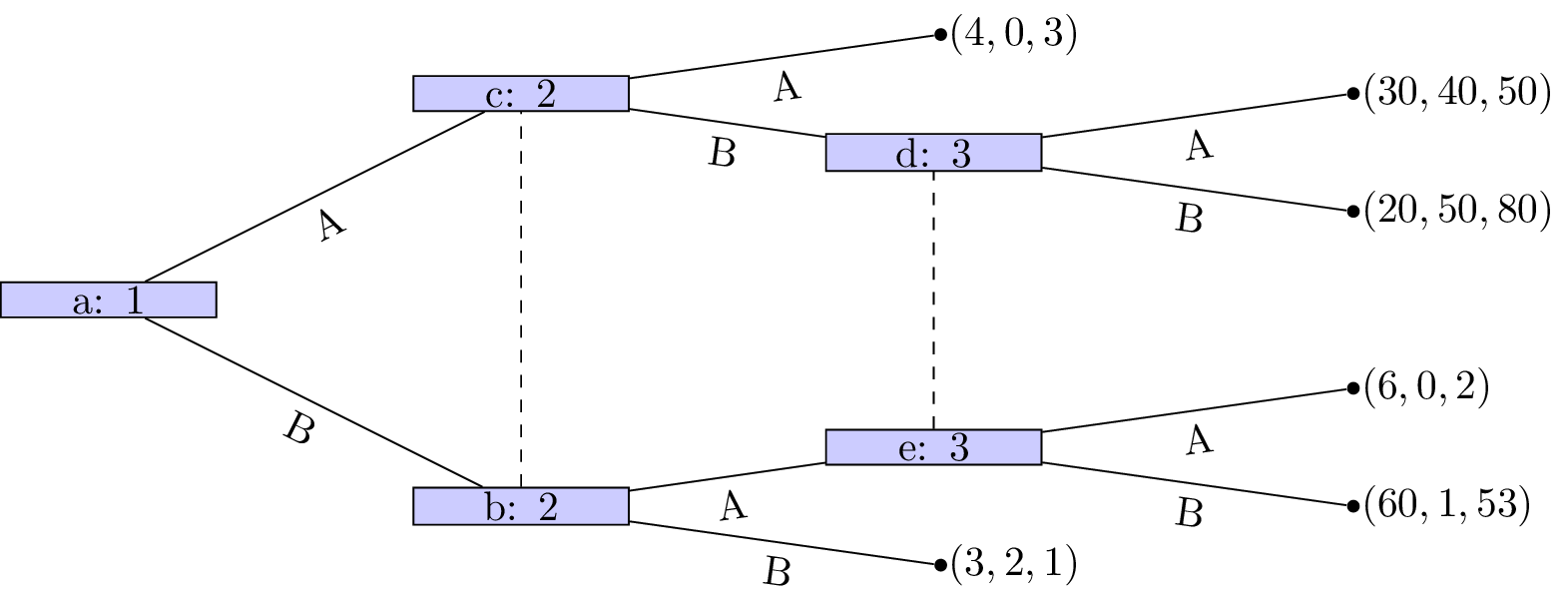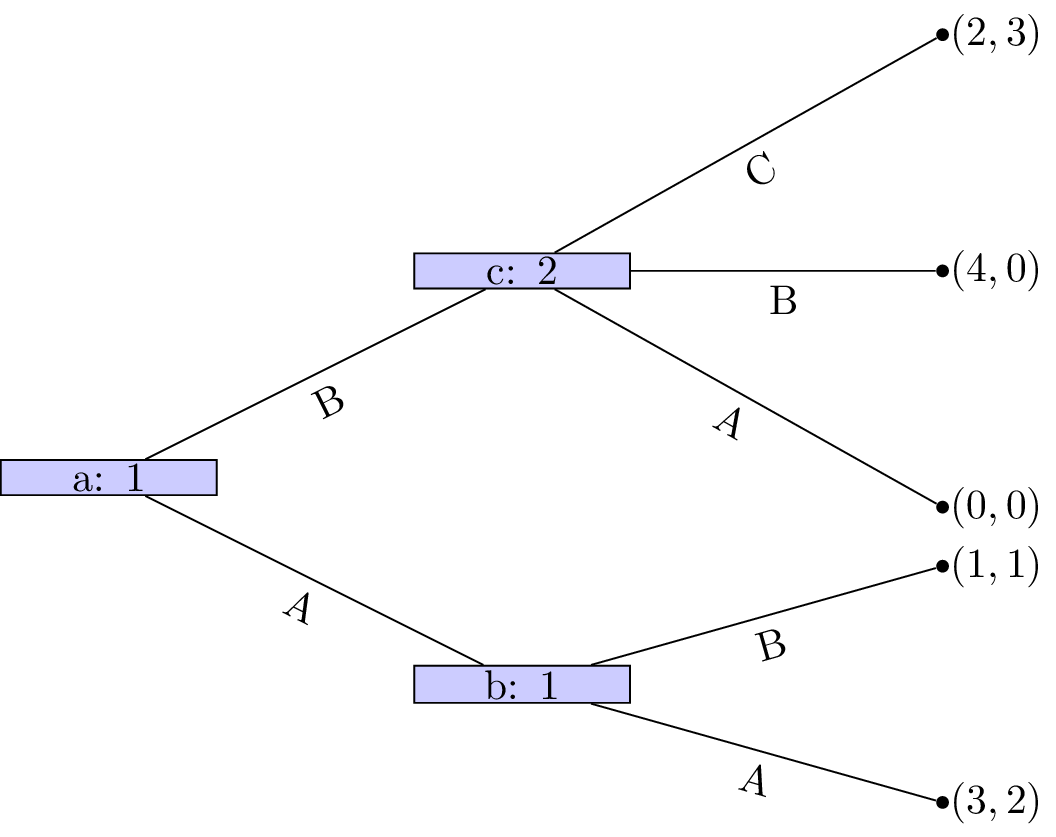2. Obtain the Nash equilibrium for the following game:

Player 1 chooses a number $$x\geq 0$$, which player 2 observes. After this simulataneously and independatly player 1 and player 2 choose $$y_1, y_2\in\mathbb{R}$$ respectively. The utility to player 1 is given by $$2y_2y_1+xy_1-y_1^2-x^3/3$$ and the utility to player 2 is given by $$-(y_1-2y_2)^2$$.

3. For each of the following games:

i. Identify all subgames. ii. Identify the corresponding normal form representations and hence obtain all Nash equilibrium. iii. Identify which Nash equilibrium are also subgame perfect Nash equilibrium.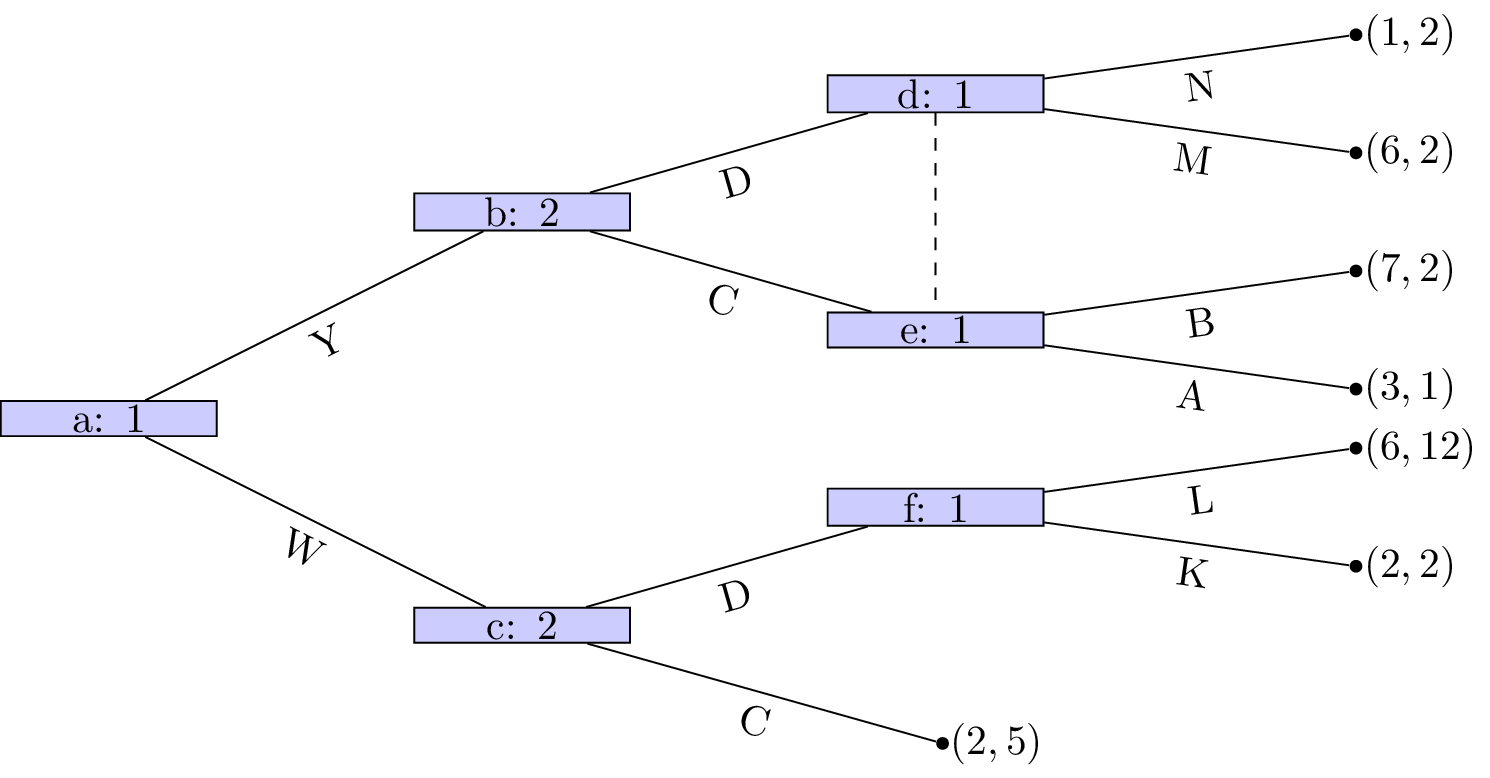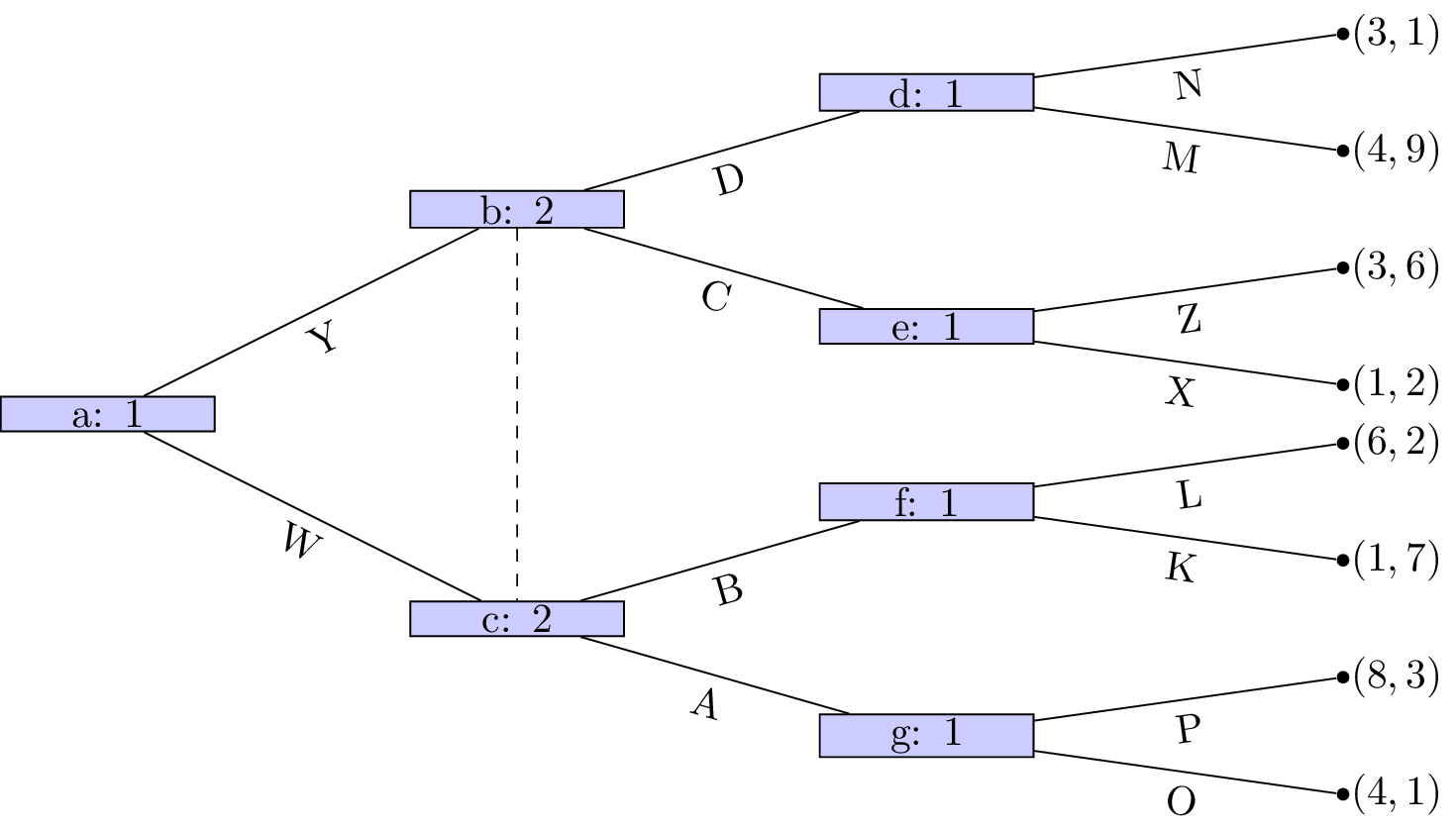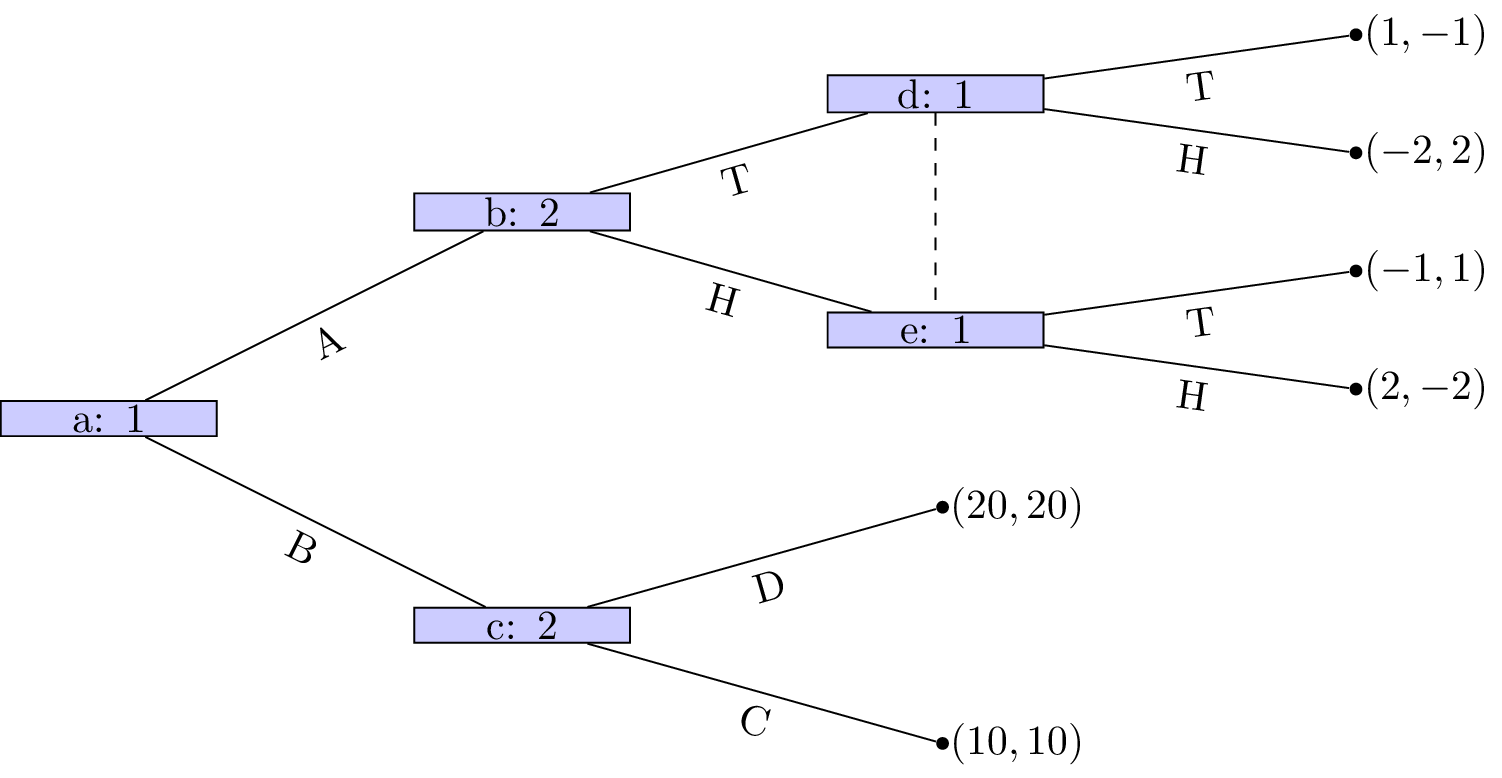4. For the following stage games:

i. Plot all possible utility pairs for $$T=2$$; ii. Recalling that subgame perfect equilibrium for the repeated game must play a stage Nash equilibrium in the final stage attempt to identify a subgame perfect Nash equilibrium for the repeated game that is not a sequence of stage Nash profiles.

5. Consider the following stage game:

i. For $$\delta=1/3$$ obtain the utilities for the infinitely repeated game for the strategies $$S_D$$: “play the first strategy throughout” and $$S_C$$: “play the second strategy throughout”. ii. Plot the space of feasible average payoffs and the space of individually rational payoffs. iii. State whether or not it is possible according to the Folk theorem to obtain $$\delta$$ that ensures that a strategy profile exists that would give a subgame perfect Nash equilibrium with average payoffs: $$(3/2,3/2)$$, $$(0,3)$$, $$(2,6)$$ and $$(2,0)$$.

Solution available